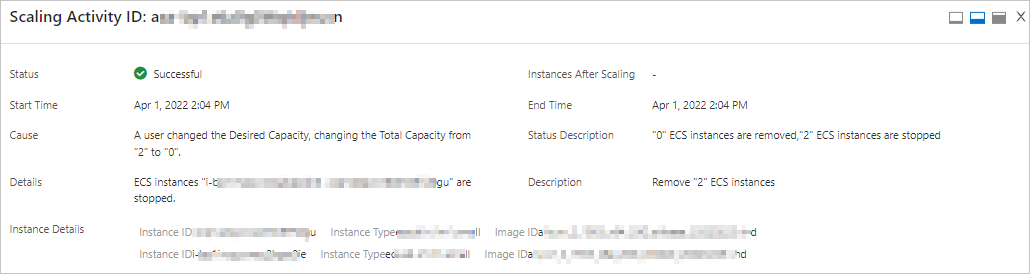This topic describes how to create a scheduled task to configure the maximum number, minimum number, and expected number of instances in a scaling group.

## Background information

Auto Scaling allows you to configure or modify the maximum number, minimum number, and expected number of instances in a scaling group by using the following methods:
• You can configure the maximum number, minimum number, and expected number of instances in a scaling group when you create the scaling group. You can also modify the maximum number, minimum number, and expected number of instances in a scaling group after you create the scaling group. For more information, see Create a scaling group and Modify a scaling group.
• You can configure the maximum number, minimum number, and expected number of instances in a scaling group when you create a scheduled task for the scaling group. You can also modify the maximum number, minimum number, and expected number of instances in the scaling group after you create the scheduled task.
When the scheduled task that you create triggers a scaling activity in a scaling group, the maximum number, minimum number, and expected number of instances in the scaling group are automatically updated to the maximum number, minimum number, and expected number of instances that you configure when you create the scheduled task. If you do not configure the maximum number, minimum number, and expected number of instances when you create a scheduled task for a scaling group, the maximum number, minimum number, and expected number of instances in the scaling group remain unchanged after the scheduled task triggers a scaling activity. The following limits apply to the maximum number, minimum number, and expected number of instances in a scaling group:
• The minimum number of instances must be smaller than or equal to the maximum number of instances.
• The minimum number of instances must be smaller than or equal to the expected number of instances.
• The expected number of instances must be smaller than or equal to the maximum number of instances.

If the preceding conditions are not met, scaling activities fail. Examples:

• Example 1: A scaling activity fails because the minimum number of instances is larger than the maximum number of instances. In this example, the maximum number of instances in the scaling group is set to 2, and the minimum number of instances in the scaling group is set to 0. When you create a scheduled task for the scaling group, you set the minimum number of instances to 3. Therefore, when the scheduled task triggers a scaling activity, the system attempts to update the minimum number of instances from 0 to 3. However, the scaling activity fails because the minimum number of instances is larger than the maximum number of instances.
• Example 2: A scaling activity fails because the minimum number of instances is larger than the expected number of instances. In this example, the maximum number of instances in the scaling group is set to 10, the minimum number of instances in the scaling group is set to 0, and the expected number of instances in the scaling group is set to 5. When you create a scheduled task for the scaling group, you set the minimum number of instances to 6. Therefore, when the scheduled task triggers a scaling activity, the system attempts to update the minimum number of instances from 0 to 6. However, the scaling activity fails because the minimum number of instances is larger than the expected number of instances.
• Example 3: A scaling activity fails because the expected number of instances is larger than the maximum number of instances. In this example, the maximum number of instances in the scaling group is set to 5, the minimum number of instances in the scaling group is set to 0, and the expected number of instances in the scaling group is set to 3. When you create a scheduled task for the scaling group, you set the expected number of instances to 6. Therefore, when the scheduled task triggers a scaling activity, the system attempts to update the expected number of instances from 3 to 6. However, the scaling activity fails because the expected number of instances is larger than the maximum number of instances.

## Precautions

• When you create or modify a scheduled task, you can specify Select Existing Scaling Rule or Configure Number of Instances in Scaling Group as the scaling method. You cannot specify both methods at the same time.
• When you create a scheduled task, you must configure at least one of the following parameters: Maximum Instances, Minimum Instances, and Expected Instances. The minimum number of instances must be smaller than or equal to the expected number of instances. The expected number of instances must be smaller than or equal to the maximum number of instances.
• If you do not configure an expected number of instances when you create a scaling group, you cannot configure the expected number of instances when you create or modify a scheduled task for the scaling group.
• For scaling groups for which an expected number of instances is configured, we recommend that you configure the maximum number, minimum number, and expected number of instances when you create scheduled tasks to ensure that the scheduled tasks can be executed.

## Procedure

1. Log on to the Auto Scaling console.
For information about how to view the execution details about scaling activities, see View the details of a scaling activity.